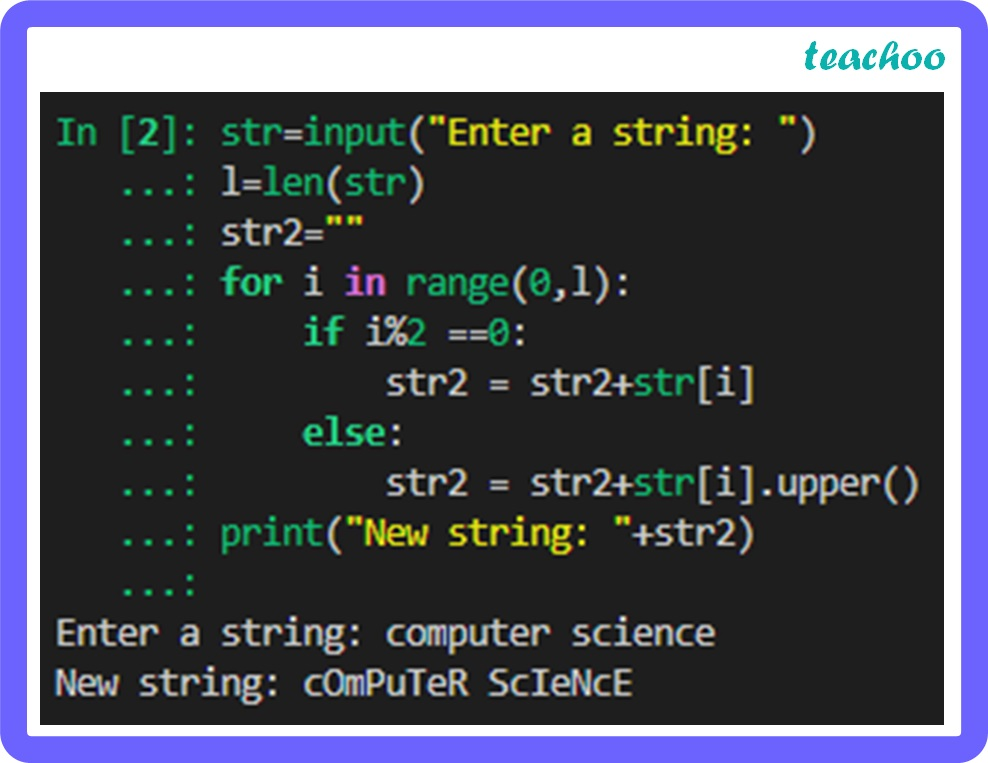Long Answer Type Questions (5 Marks each)

Computer Science - Class 11
Chapter 8 Class 11 - Strings

## Write a program that reads a string and then prints a string that capitalizes every other letter in the string, for example computer becomes cOmPuTeR.Code:

``` str=input( "Enter a string: " ) ```

``` l=len(str) ```

``` str2= "" ```

``` for i in range( 0 ,l): ```

```     if i% 2 == 0 : ```

```         str2 = str2+str[i] ```

```     else: ```

```         str2 = str2+str[i].upper() ```

``` print("New string: "+str2) ```

Explanation:

upper( ) is an in-built method used to convert lowercase letters to uppercase.

Learn in your speed, with individual attention - Teachoo Maths 1-on-1 Class